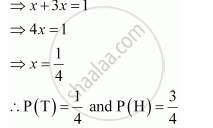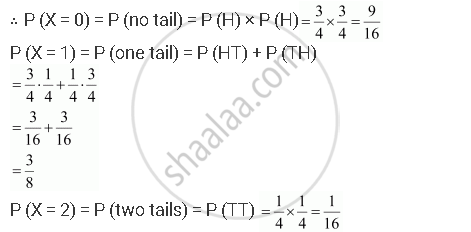Share

# A Coin is Biased So that the Head is 3 Times as Likely to Occur as Tail. If the Coin is Tossed Twice, Find the Probability Distribution of Number of Tails. - CBSE (Science) Class 12 - Mathematics

ConceptRandom Variables and Its Probability Distributions

#### Question

A coin is biased so that the head is 3 times as likely to occur as tail. If the coin is tossed twice, find the probability distribution of number of tails.

#### Solution

Let the probability of getting a tail in the biased coin be x.

∴ P (T) = x

⇒ P (H) = 3x

For a biased coin, P (T) + P (H) = 1When the coin is tossed twice, the sample space is {HH, TT, HT, TH}.

Let X be the random variable representing the number of tails.Therefore, the required probability distribution is as follows.

 X 0 1 2 P(X) 9/16 3/8 1/16
Is there an error in this question or solution?

#### Video TutorialsVIEW ALL 

Solution A Coin is Biased So that the Head is 3 Times as Likely to Occur as Tail. If the Coin is Tossed Twice, Find the Probability Distribution of Number of Tails. Concept: Random Variables and Its Probability Distributions.
S